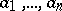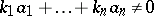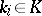# Linear independence

(diff) ← Older revision | Latest revision (diff) | Newer revision → (diff)

One of the main concepts in linear algebra. Letbe a vector space over a field; the vectorsare said to be linearly independent iffor any setexcept. Otherwise the vectors() are said to be linearly dependent. The vectorsare linearly dependent if and only if at least one of them is a linear combination of the others. An infinite subset of vectors ofis said to be linearly dependent if some finite subset of it is linearly dependent, and linearly independent if any finite subset of it is linearly independent. The number of elements (the cardinality) of a maximal linearly independent subset of a space does not depend on the choice of this subset and is called the rank, or dimension, of the space, and the subset itself is called a basis (or base).

In the special case when the vectorsare elements of some number fieldandis a subfield of, there arises the concept of linear independence of numbers. Linear independence of numbers over the field of rational numberscan be regarded as a generalization of the concept of irrationality (cf. Irrational number). Thus, the two numbersandare linearly independent if and only ifis irrational.

The concepts of linear dependence and independence of elements have also been introduced for Abelian groups and modules.

Linear dependence is a special case of a wider concept, that of an abstract dependence relation on a set.